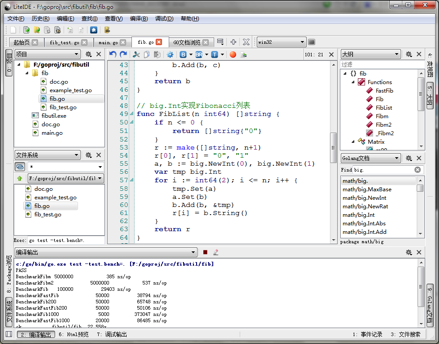# Go 语言开发工具 LiteIDE

categories:资料  author:

Go 语言最初在 2009 年 11 月对外公布，在 2011 年 3 月 16 日发布第一个 release，第一个正式版本 Go1 于 2012 年 3 月 28 日推出。在 Go 语言的正式版本推出后，Eclipse、IntelliJ IDEA、vim、emacs、gedit、SublimeText2、Textmate、Textpad、SciTE、Notepad++ 等 IDE 和编辑器开始纷纷有了各自的 Go 语言插件。

LiteIDE 是一款专为 Go 语言开发而设计的跨平台轻量级集成开发环境（IDE），基于 Qt 开发，支持 Windows、Linux 和 Mac OS X 平台。LiteIDE 的第一个版本发布于 2011 年 1 月初，是最早的面向 Go 语言的 IDE 之一。到 2013 年 1 月为止，LiteIDE 已经发布到版本 X16。

## LiteIDE 主要特点

• 支持主流操作系统
• Windows
• Linux
• MacOS X
• Go 编译环境管理和切换
• 管理和切换多个 Go 编译环境
• 支持 Go 语言交叉编译
• 与 Go 标准一致的项目管理方式
• 基于 GOPATH 的包浏览器
• 基于 GOPATH 的编译系统
• 基于 GOPATH 的 Api 文档检索
• Go 语言的编辑支持
• 类浏览器和大纲显示
• Gocode(代码自动完成工具) 的完美支持
• Go 语言文档查看和 Api 快速检索
• 代码表达式信息显示 F1
• 源代码定义跳转支持 F2
• Gdb 断点和调试支持
• gofmt 自动格式化支持
• 其他特征
• 支持多国语言界面显示
• 完全插件体系结构
• 支持编辑器配色方案
• 基于 Kate 的语法显示支持
• 基于全文的单词自动完成
• 支持键盘快捷键绑定方案
• Markdown 文档编辑支持
• 实时预览和同步显示
• 自定义 CSS 显示
• 可导出 HTML 和 PDF 文档
• 批量转换 / 合并为 HTML/PDF 文档

## 安装配置

```GOROOT=c:\go
GOBIN=
GOARCH=amd64
GOOS=windows
CGO_ENABLED=1

PATH=%GOBIN%;%GOROOT%\bin;%PATH%
。。。```

```GOROOT=\$HOME/go
GOBIN=
GOARCH=amd64
GOOS=linux
CGO_ENABLED=1

PATH=\$GOBIN:\$GOROOT/bin:\$PATH
。。。```

## 使用 LiteIDE 开发一个简单的 Go 语言应用示例

### 项目简介### Fibonacci 数列规律

```0 1 1 2 3 5 8 13 21 34 55 89 144 ...
... -21 13 -8 5 －3 2 －1 1 0 1 1 2 3 5 8 13 21 …```

### 编写 fib 函数

```// 非递归方式实现 Fibonacci 算法
func Fibm(n int64) int64 {
if n == 0 {
return 0
}
var i, a, b int64
b = 1
if n < 0 {
n = -n
if n%2 == 0 {
b = -1
}
}
for i = 2; i <= n; i++ {
a, b = b, a+b
}
return b
}

// 递归方式实现 Fibonacci 算法
func Fibm2(n int64) int64 {
if n < 0 {
if n%2 == 0 {
return _Fibm2(-n, 0, -1)
} else {
return _Fibm2(-n, 0, 1)
}
}
return _Fibm2(n, 0, 1)
}

func _Fibm2(n, a1, a2 int64) int64 {
if n == 0 {
return a1
}
return _Fibm2(n-1, a2, a1+a2)
}```

### 编写测试

```    package fib         // 称为 Test 方式，可以直接使用 fib 包内函数
package fib_test    // 称为 XTest 方式, 因为包名与 fib 不同，必须使用 import "fibutil/fib"

```

```package fib

import (
"testing"
)

func TestFibm(t *testing.T) {
ar := []int64{0, 1, 1, 2, 3, 5, 8, 13, 21}
for i := 0; i < len(ar); i++ {
if ar[i] != Fibm(int64(i)) {
t.Fatalf("%d, %d != %d", i, ar[i], Fibm(int64(i)))
}
}
ar1 := []int64{0, 1, -1, 2, -3, 5, -8, 13, -21}
for i := 0; i < len(ar1); i++ {
if ar1[i] != Fibm(int64(-i)) {
t.Fatalf("%d, %d != %d", -i, ar1[i], Fibm(int64(-i)))
}
}
}

func TestFibm2(t *testing.T) {
。。。```

LiteIDE 在保存时会自动格式化源码，现在点击工具栏上的测试按钮（Ctrl+T）进行测试（等同于 go test），如果测试通过则显示

```PASS
ok      fibutil/fib 0.036s```

```--- FAIL: TestFibm (0.00 seconds)
fib_test.go:11: 8, 22 != 21
FAIL
exit status 1
FAIL    fibutil/fib 0.035s```

### 性能测试

```func BenchmarkFibm(b *testing.B) { for i := 0; i < b.N; i++ { Fibm(90) } }
func BenchmarkFibm2(b *testing.B) {
。。。```

```PASS
BenchmarkFibm    5000000           358 ns/op
BenchmarkFibm2   5000000           513 ns/op
ok      fibutil/fib 5.286s```

### 支持大数操作

```math/big
math/big.NewInt
math/big.Int
math/big.Abs
。。。```

```// big.Int 实现 Fibonacci 算法
func Fib(n int64) *big.Int {
if n == 0 {
return big.NewInt(0)
}
a, b, c := big.NewInt(0), big.NewInt(1), big.NewInt(0)
if n < 0 {
n = -n
if n%2 == 0 {
b.SetInt64(-1)
}
}
for i := int64(2); i <= n; i++ {
c.Set(a)
a.Set(b)
}
return b
}

// 使用矩阵求解 Fibonacci 算法
func FastFib(n int64) *big.Int {
。。。

// big.Int 实现 Fibonacci 列表
func FibList(n1,n2 int64) []string {
。。。```

```PASS
BenchmarkFibm    5000000           359 ns/op
BenchmarkFibm2   5000000           513 ns/op
BenchmarkFib      100000         28878 ns/op
BenchmarkFastFib       50000         36354 ns/op
BenchmarkFib200    50000         65398 ns/op
BenchmarkFastFib200    50000         47926 ns/op
BenchmarkFib1000        5000        350344 ns/op
BenchmarkFastFib1000       20000         78159 ns/op
ok      fibutil/fib 21.607s```

### 编写 Fib 函数代码示例

```package fib

import (
"fmt"
)

func ExampleFibList() {
fmt.Println(FibList(-10, 10))
// Output: [-55 34 -21 13 -8 5 -3 2 -1 1 0 1 1 2 3 5 8 13 21 34 55]
}```

### 编写 fibutil 主程序

```package main

import (
"fibutil/fib"
"fmt"
"os"
"strconv"
)

func main() {
switch len(os.Args) {
case 2:
n, err := strconv.ParseInt(os.Args, 10, 64)
if err == nil {
fmt.Println(fib.FastFib(n))
return
}
case 3:
n1, e1 := strconv.ParseInt(os.Args, 10, 64)
n2, e2 := strconv.ParseInt(os.Args, 10, 64)
if e1 == nil && e2 == nil {
fmt.Println(fib.FibList(n1, n2))
return
}
}
fmt.Fprintf(os.Stderr, "%s, fibonacci number util\n\tfibutil n\t:fibonacci number\n\tfibutil n1 n2\t:fibonacci number list\n", os.Args)
}```

`[-55 34 -21 13 -8 5 -3 2 -1 1 0 1 1 2 3 5 8 13 21 34 55]`

### 调试

Go 语言编译器 gc 生成 DWARF 格式调试信息，gdb7.1 以上可以很好的调试 Go 语言，LiteIDE 集成了图形化调试功能，提供断点设置、显示变量值、函数调用栈、添加变量监视等功能，同时支持调试控制台直接输入 gdb 调试命令。在对 Go 源程序调试时，如果需要禁用优化和内联，可以使用工具栏编译配置－编译自定义－BUILDARGS 中输入 -gcflags “-N -l” 重新编译后调试即可，在菜单－选项－LiteDebug 中可以选择调试前重编译，这样每次调试时会自动重新编译。

### 交叉编译

Go 语言支持多平台交叉编译，我们准备将 fibutil 交叉编译为 Linux-amd64 目标。首先要编译 Go 源码以创建目标平台所需要的包和工具, 进入 cmd 命令行执行:

```> set GOOS=linux
> set GOARCH=amd64
> set CGO_ENABLED=0
> cd c:\go\src
> all.bat```

### 本文代码

fibutil 源代码可以从https://github.com/visualfc/fibutil 下载，可以使用 go get 安装: go get -v github.com/visualfc/fibutil。 github 网站上的代码与本文示例代码区别在于于引用位置不同，即在 fibutil.go 文件中使用 import github.com/visualfc/fibutil/fib 替代 import fibutil/fib

## 关于作者

visualfc，非计算机专业毕业的狂热程序员，擅长 C++、Lua、Go 等语言，热衷于开源软件，业余时间开发了 WTL 可视化开发插件 VisualFC(http://code.google.com/p/visualfc)、基于 Qt 的跨平台轻量级 Go 语言集成开发环境 LiteIDE(http://code.google/p/golangide)，他的邮件是 visualfc@gmail.com。